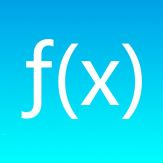English

Every day we offer FREE licensed iOS apps and games you’d have to buy otherwise.\$0.99
free today

# iPhone Giveaway of the Day - Calculator Advanced

The Fx saves your formulas and then you can easily do the calculations you want using these formulas.
\$0.99 free today

is available as a Giveaway of the day!Calculator Advanced: The Fx saves your formulas and then you can easily do the calculations you want using these formulas. After you save your formula, YOU DO NOT NEED TO REWRITE YOUR FORMULA. ONLY IT IS ENOUGH TO ENTER THE VARIABLES. So you can use the formula you want over and over again.

Features
*Supported sine, cosine, tangent, cotangent, arcsine, arccosine, arctangent,
arccotangent, absolute value, logarithm, natural logarithm, combination,
permutation, factorial

*Supported list variable. You can calculate sum of the elements,
number of elements, Multiply all numbers, Median,
Sum of the inverse of the elements by multiplication, standard deviation

*Built-in 84 formulas

*With Scientific Calculator with Math keyboard

*With User Manual

Mert Can Kus

Productivity

1.4

1.52 MB

4+

English

### Compatibility: